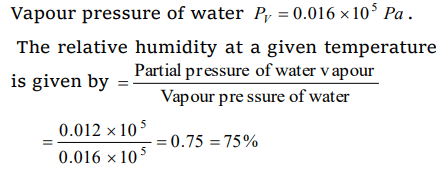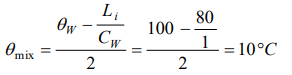## Thermal Properties of Matter Questions and Answers Part-13

1. One kilogram of ice at 0°C is mixed with one kilogram of water at 80°C. The final temperature of the mixture is ( Take : specific heat of water = $4200 J kg^{-1}k^{-1}$   , latent heat of ice = $336 KJkg^{-1}$   )
a) 40°C
b) 60°C
c) 0°C
d) 50°C

Explanation:2. During constant temperature, we feel colder on a day when the relative humidity will be
a) 25%
b) 12.5%
c) 50%
d) 75%

Explanation: When the relative humidity is low (approx. 25%), the evaporation from our body is faster. Thus we feel colder

3. Which of the following is the unit of specific heat
a) $J kg^{\circ}C^{-1}$
b) $J / kg^{\circ}C$
c) $kg^{\circ}C / J$
d) $J / kg^{\circ}C^{-2}$

Explanation:4. 50 gm of ice at 0°C is mixed with 50 gm of water at 80°C, final temperature of mixture will be
a) 0°C
b) 40°C
c) 80°C
d) 4°C

Explanation:5. The freezing point of the liquid decreases when pressure is increased, if the liquid
a) Expands while freezing
b) Contracts while freezing
c) Does not change in volume while freezing
d) None of these

Explanation: Freezing point of water decreases when pressure increases, because water expands on solidification while “except water” for other liquid freezing point increases with increase in pressure. Since the liquid in question is water. Hence, it expands on freezing

6. The relative humidity on a day, when partial pressure of water vapour is $0.012\times 10^{5}Pa$    at 12°C is (take vapour pressure of water at this temperature as $0.016\times 10^{5}Pa$   )
a) 70%
b) 40%
c) 75%
d) 25%

Explanation: Partial pressure of water vapour Pw = 0.012 * 105 Pa,7. A hammer of mass 1kg having speed of 50 m/s, hit a iron nail of mass 200 gm. If specific heat of iron is 0.105 cal/gm°C and half the energy is converted into heat, the raise in temperature of nail is
a) 7.1°C
b) 9.2°C
c) 10.5°C
d) 12.1°C

Explanation:8. Latent heat of 1gm of steam is 536 cal/gm, then its value in joule/kg is
a) $2.25\times 10^{6}$
b) $2.25\times 10^{3}$
c) 2.25
d) None

Explanation:9. Which of the following has maximum specific heat
a) Water
b) Alcohol
c) Glycerine
d) Oil